#### 期刊菜单

A Description of Negative Frequency and Phase Angle by Rotating Vector
DOI: 10.12677/OJCS.2020.93007, PDF, HTML, XML, 下载: 359  浏览: 1,160  科研立项经费支持

Abstract: In some teaching materials of signals and systems, negative frequency is said to have no physical meaning, and the definition of phase angle is not strict enough. In this paper, the negative fre-quency and phase angle are described by the method of rotation vector. From the geometric sense, if the positive frequency is the counter clockwise rotation angular velocity of the vector, then the negative frequency is the angular velocity of clockwise rotation. From the engineering point of view, it can correspond to the reverse rotation of the generator. Therefore, negative frequency not only has clear physical significance, but also has important engineering application values. In general, the phase angle is defined by the arctangent trigonometric function, but the range of the phase angle is −π/2 to π/2, the actual phase angle should be −π to π. This problem can be strictly defined by rotation vector.

1. 引言

2. 傅里叶级数的描述

2.1. 三角形式的傅里叶级数

$\begin{array}{l}x\left(t\right)={a}_{0}+\underset{n=1}{\overset{\infty }{\sum }}{a}_{n}\mathrm{cos}\left(n{\omega }_{0}t\right)+{b}_{n}\mathrm{sin}\left(n{\omega }_{0}t\right)\\ {a}_{0}=\frac{1}{T}{\int }_{{t}_{0}}^{{t}_{0}+T}x\left(t\right)\text{d}t\\ {a}_{n}=\frac{2}{T}{\int }_{{t}_{0}}^{{t}_{0}+T}x\left(t\right)\mathrm{cos}\left(n{\omega }_{0}t\right)\text{d}t\\ {b}_{n}=\frac{2}{T}{\int }_{{t}_{0}}^{{t}_{0}+T}x\left(t\right)\mathrm{sin}\left(n{\omega }_{0}t\right)\text{d}t\end{array}$ (1)

$\begin{array}{l}x\left(t\right)={a}_{0}+\underset{n=1}{\overset{\infty }{\sum }}{A}_{n}\left[\mathrm{cos}\left(n{\omega }_{0}t+{\phi }_{n}\right)\right]\\ {A}_{n}=\sqrt{{a}_{n}^{2}+{b}_{n}^{2}}\\ {\phi }_{n}=-\mathrm{arctan}\left({b}_{n}/{a}_{n}\right)\end{array}$ (2)

2.2. 指数形式的傅里叶级数

$\begin{array}{l}x\left(t\right)=\underset{n=-\infty }{\overset{\infty }{\sum }}{c}_{n}{\text{e}}^{jn{\omega }_{0}t}\\ {c}_{n}=\frac{1}{T}{\int }_{{t}_{0}}^{{t}_{0}+T}x\left(t\right){\text{e}}^{-jn{\omega }_{0}t}\text{d}t=\frac{1}{2}{\stackrel{˙}{A}}_{n}{\text{e}}^{j{\phi }_{n}}=\frac{1}{2}\left({a}_{n}-j{b}_{n}\right)\end{array}$ (3)

3. 旋转矢量的定义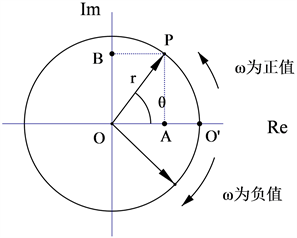Figure 1. Rotation vector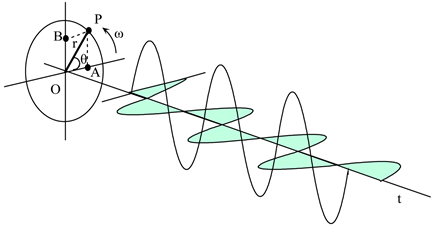Figure 2. Rotation vector and complex sine wave

$r{\text{e}}^{j\left(\omega t+\theta \right)}=r\left[\mathrm{cos}\left(\omega t+\theta \right)+j\mathrm{sin}\left(\omega t+\theta \right)\right]$ (4)

$r{\text{e}}^{j\left(\omega t+\theta \right)}$ 是简单的复正弦波，其立体图不易表达，所以一般用两张图来表示，如图3所示。Figure 3. Real part and imaginary part of complex sine wave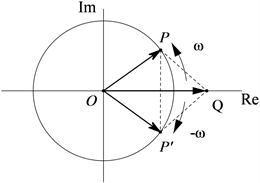Figure 4. The superposition of two conjugate complex sinusoids forms a real sine wave

$\mathrm{cos}\left(\omega t\right)=\frac{{\text{e}}^{j\omega t}+{\text{e}}^{-j\omega t}}{2}$, $\mathrm{sin}\left(\omega t\right)=\frac{{\text{e}}^{j\omega t}-{\text{e}}^{-j\omega t}}{2j}$

4. 用旋转矢量解释傅里叶级数中的负频率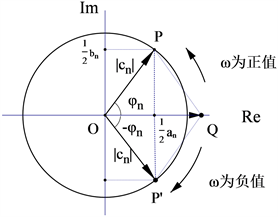Figure 5. The rotation vector is used to express the parameters of Fourier series

$v=\frac{\text{d}s}{\text{d}t}$, $\omega =\frac{\text{d}\theta }{\text{d}t}$

5. 用旋转矢量定义相位角

${\phi }_{n}=-\mathrm{arctan}\left(\frac{{b}_{n}}{{a}_{n}}\right)$$\mathrm{tan}{\phi }_{n}=-\frac{{b}_{n}}{{a}_{n}}$ (5)

$\mathrm{cos}\left({\omega }_{0}t\right)+\mathrm{sin}\left({\omega }_{0}t\right)=\sqrt{2}\mathrm{cos}\left({\omega }_{0}t+\frac{\pi }{4}\right)$

$-\mathrm{cos}\left({\omega }_{0}t\right)-\mathrm{sin}\left({\omega }_{0}t\right)=\sqrt{\text{2}}\mathrm{cos}\left({\omega }_{0}t+\frac{5\pi }{4}\right)$ (6)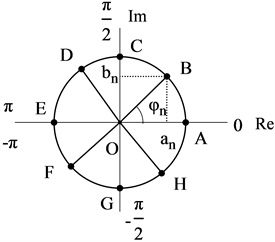Figure 6. Relationship between phase angle φn and an, bn

A： ${a}_{n}>0$${b}_{n}=0$${\phi }_{n}=0$ B： ${a}_{n}>0$${b}_{n}>0$$0<{\phi }_{n}<\pi /2$

C： ${a}_{n}=0$${b}_{n}>0$${\phi }_{n}=\pi /2$ D： ${a}_{n}<0$${b}_{n}>0$$\pi /2<{\phi }_{n}<\pi$

E： ${a}_{n}<0$${b}_{n}=0$${\phi }_{n}=\pi$ F： ${a}_{n}<0$${b}_{n}<0$$-\pi <{\phi }_{n}<-\pi /2$

G： ${a}_{n}=0$${b}_{n}<0$${\phi }_{n}=-\pi /2$ H： ${a}_{n}>0$${b}_{n}<0$$-\pi /2<{\phi }_{n}<0$

O： ${a}_{n}=0$${b}_{n}=0$，φn取任意值

${\phi }_{n}=\mathrm{arg}\left({a}_{n},{b}_{n}\right)$ (7)

${\phi }_{n}=\mathrm{arg}\left({c}_{n}\right)$ (8)

6. 结论

NOTES

*通讯作者。

  郑君里, 应启珩, 杨为理. 信号与系统[M]. 第二版. 北京: 高等教育出版社, 2000.  王宝祥. 信号与系统[M]. 第三版. 北京: 电子工业出版社, 2010.  陈怀琛, 方海燕. 论频谱中负频率成分的物理意义[J]. 电气电子教学学报, 2008, 30(1): 29-32.  张金平, 李建立, 段晨. 计及负频率影响的新能源发电低频间谐波检测方法[J]. 电测与仪表, 2020, 57(2): 95-100.  刘型志, 周全, 张淮清, 田娟, 陈文礼. 基于负频率频谱干扰消除的高精度低频间谐波检测算法[J]. 重庆大学学报, 2020, 43(2): 50-59.